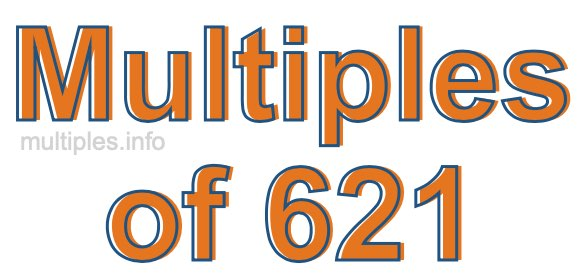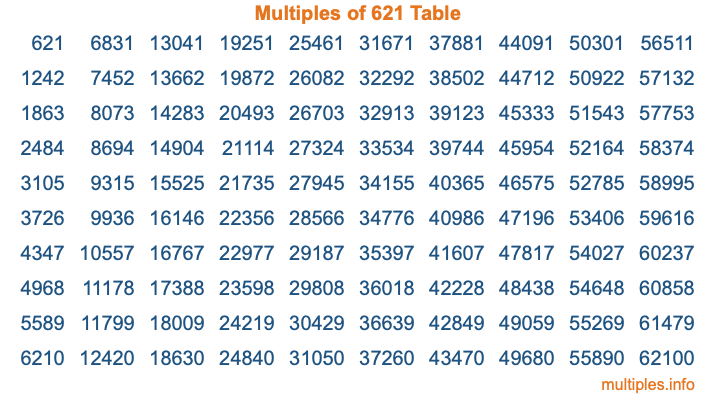Multiples of 621Welcome to the Multiples of 621 page. Here we will first teach you everything you will ever need to know about the multiples of 621, and then give you a study guide summary of everything we taught you to make sure you remember it all. Use this page to look up facts and learn information about the multiples of 621. This page will make you a multiples of six hundred twenty-one expert!

Definition of Multiples of 621
Multiples of 621 are all the numbers that when divided by 621 equal an integer. Each of the multiples of 621 are called a multiple. A multiple of 621 is created by multiplying 621 by an integer.

Therefore, to create a list of multiples of 621, you start with 1 multiplied by 621, then 2 multiplied by 621, then 3 multiplied by 621, and so on for as long as you want. Thus, the list of the first five multiples of 621 is 621, 1242, 1863, 2484, and 3105. To see a larger list of multiples of 621, see the printable image of Multiples of 621 further down on this page. We also have a category where you can choose any nth multiple of 621.

Multiples of 621 Checker
The Multiples of 621 Checker below checks to see if any number of your choice is a multiple of 621. In other words, it checks to see if there is any number (integer) that when multiplied by 621 will equal your number. To do that, we divide your number by 621. If the the quotient is an integer, then your number is a multiple of 621.

Is  a multiple of 621?

Least Common Multiple of 621 and ...
A Least Common Multiple (LCM) is the lowest multiple that two or more numbers have in common. This is also called the smallest common multiple or lowest common multiple and is useful to know when you are adding our subtracting fractions. Enter one or more numbers below (621 is already entered) to find the LCM.

Check out our LCM Calculator if you need more details about the Least Common Multiple or if you need the LCM for different numbers for adding and subtraction fractions.

nth Multiple of 621
As we stated above, 621 is the first multiple of 621, 1242 is the second multiple of 621, 1863 is the third multiple of 621, and so on. Enter a number below to find the nth multiple of 621.

th multiple of 621

Multiples of 621 vs Factors of 621
621 is a multiple of 621 and a factor of 621, but that is where the similarities end. All postive multiples of 621 are 621 or greater than 621. All positive factors of 621 are 621 or less than 621.

Below is the beginning list of multiples of 621 and the factors of 621 so you can compare:

Multiples of 621: 621, 1242, 1863, 2484, 3105, etc.

Factors of 621: 1, 3, 9, 23, 27, 69, 207, 621

As you can see, the multiples of 621 are all the numbers that you can divide by 621 to get a whole number. The factors of 621, on the other hand, are all the whole numbers that you can multiply by another whole number to get 621.

It's also interesting to note that if a number (x) is a factor of 621, then 621 will also be a multiple of that number (x).

Multiples of 621 vs Divisors of 621
The divisors of 621 are all the integers that 621 can be divided by evenly. Below is a list of the divisors of 621.

Divisors of 621: 1, 3, 9, 23, 27, 69, 207, 621

The interesting thing to note here is that if you take any multiple of 621 and divide it by a divisor of 621, you will see that the quotient is an integer.

Multiples of 621 Table
Below is an image of the first 100 multiples of 621 in a table. The table is in chronological order, column by column. The first column has the first ten multiples of 621, the second column has the next ten multiples of 621, and so on.The Multiples of 621 Table is also referred to as the 621 Times Table or Times Table of 621. You are welcome to print out our table for your studies.

Negative Multiples of 621
Although not often discussed or needed in math, it is worth mentioning that you can make a list of negative multiples of 621 by multiplying 621 by -1, then by -2, then by -3, and so on, to get the following list of negative multiples of 621:

-621, -1242, -1863, -2484, -3105, etc.

Multiples of 621 Summary
Below is a summary of important Multiples of 621 facts that we have discussed on this page. To retain the knowledge on this page, we recommend that you read through the summary and explain to yourself or a study partner why they hold true.

There are an infinite number of multiples of 621.

A multiple of 621 divided by 621 will equal a whole number.

621 divided by a factor of 621 equals a divisor of 621.

The nth multiple of 621 is n times 621.

The largest factor of 621 is equal to the first positive multiple of 621.

621 is a multiple of every factor of 621.

621 is a multiple of 621.

A multiple of 621 divided by a divisor of 621 equals an integer.

621 divided by a divisor of 621 equals a factor of 621.

Any integer times 621 will equal a multiple of 621.

Multiples of a Number
Here you can get the multiples of another number, all with the same attention to detail as we did for multiples of 621 on this page.

Multiples of
Multiples of 622
Did you find our page about multiples of six hundred twenty-one educational? Do you want more knowledge? Check out the multiples of the next number on our list!